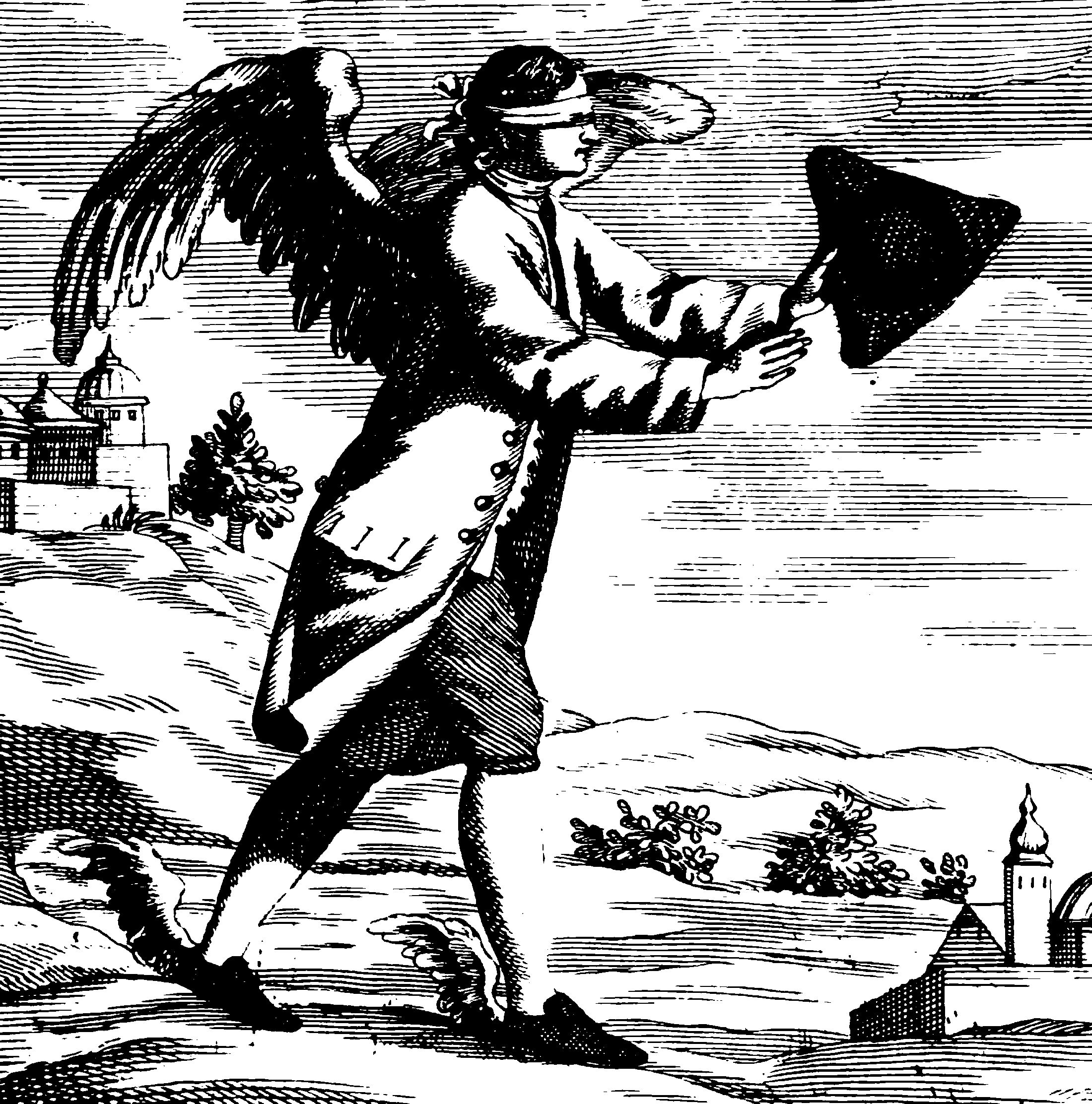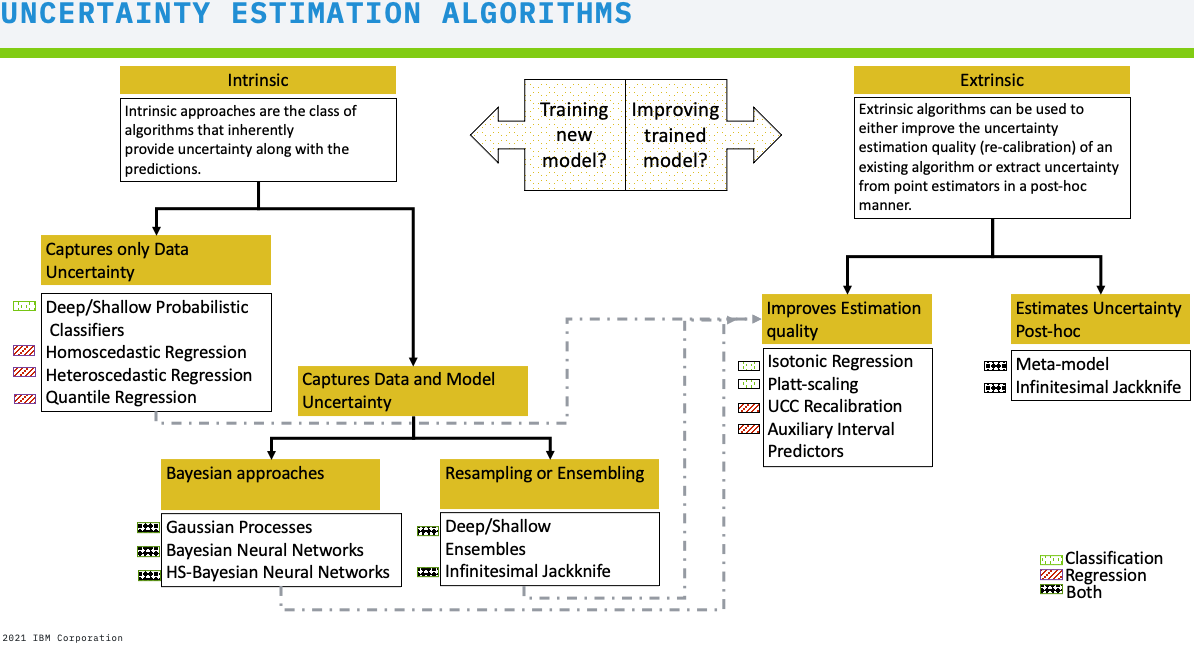# Uncertainty quantification

Using machine learning to make predictions, with a measure of the confidence of those predictions.## Taxonomy

Should clarify. TBD. Here is a recent reference on the theme: Kendall and Gal (2017) This extricates aleatoric and epistemic uncertainty. Also to mention, model uncertainty.

## DUQ networks

;Kendall and Gal (2017)

## Bayes

Bayes methods have some ideas of uncertainty baked in. You can get some way with e.g., e.g. Gaussian process regression, or probabilistic NNs.

## Physical model calibration

PEST, PEST++, and pyemu are some integrated systems for uncertainty quantification that use some weird terminology, such a FOSM (First-order-second-moment) models. I think these are best considered as inverse problem solvers, and the uncertainty quantification is a side effect of the inversion.

## Chaos expansions

See chaos expansions.

## Uncertainty Quantification 360

IBM’s Uncertainty Quantification 360 toolkit a summary of popular generic methods:

• Auxiliary Interval Predictor

Use an auxiliary model to improve the calibration of UQ generated by the original model.

• Blackbox Metamodel Classification

Extract confidence scores from trained black-box classification models using a meta-model.

• Blackbox Metamodel Regression

Extract prediction intervals from trained black-box regression models using a meta-model.

• Classification Calibration

Post-hoc calibration of classification models using Isotonic Regression and Platt Scaling.

• Heteroscedastic Regression

Train regression models that capture data uncertainty, assuming the targets are noisy and the amount of noise varies between data points.

• Homoscedastic Gaussian Process Regression

Train Gaussian Process Regression models with homoscedastic noise that capture data and model uncertainty.

• Horseshoe BNN classification

Train Bayesian neural networks classifiers with Gaussian and Horseshoe priors that capture data and model uncertainty.

• Horseshoe BNN regression

Train BNNs regression models with Gaussian and Horseshoe priors that capture data and model uncertainty.

• Infinitesimal Jackknife

Extract uncertainty from trained models by approximating the effect of training data perturbations on the model’s predictions.

• Quantile Regression

Train Quantile Regression models that capture data uncertainty, by learning two separate models for the upper and lower quantile to obtain the prediction intervals.

• UCC Recalibration

Recalibrate UQ of a regression model to specified operating point using Uncertainty Characteristics Curve

They provide guidance on method selection in the manual:Source: UQ360

### No comments yet. Why not leave one?

GitHub-flavored Markdown & a sane subset of HTML is supported.